# Three points

Mark three points E, F and G in the plane not lie on one line.

a) Draw a line segment FG
b) Construct halfline (ray) EG
c) Draw a line EF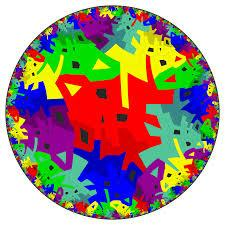Did you find an error or inaccuracy? Feel free to write us. Thank you!## Related math problems and questions:

• Draw it!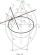Draw two lines c, d that c || d. On line c, mark the points A, B. By point A, a lead perpendicular line to c. By point B, lead perpendicular line to c.
• Straight lines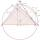Draw two lines c, d so that c || d. On line c mark points A, B, from point A start perpendicular to line c, from point B perpendicular to line c.
• Square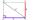Draw a square on the edge of a = 4 cm. Mark the center of symmetry S and all axes of symmetry. How many axes of symmetry does? Write down.
• LinesHow many lines can be draw with 8 points, if three points lie on one line and the other any three points do not lie on the same line?
• Line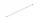How many parts of line divide 5 (different) points that lie on it?
• Points in planeThe plane is given 12 points, 5 of which is located on a straight line. How many different lines could by draw from this points?
• Square ABCDConstruct a square ABCD with cente S [3,2] and the side a = 4 cm. Point A lies on the x-axis. Construct square image in the displacement given by oriented segment SS'; S` [-1 - 4].
• Draw a trapezoidDraw a trapezoid if given a = 7 cm, b = 4 cm, c = 3.5 cm, diagonal AC = 5cm. Solve as a construction task.
• RectangleDraw a rectangle with the sides a = 4 cm, b = 5 cm. Mark the center of symmetry S and all axes of symmetry. How many axes of symmetry has? Write down.
• The sum graphically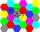Draw a graphically sum of the all sides of 4-gon ABCD.
• Points in space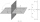There are n points, of which no three lie on one line and no four lies on one plane. How many planes can be guided by these points? How many planes are there if there are five times more than the given points?
• MO - trianglesOn the AB and AC sides of the triangle ABC lies successive points E and F, on segment EF lie point D. The EF and BC lines are parallel and is true this ratio FD:DE = AE:EB = 2:1. The area of ABC triangle is 27 hectares and line segments EF, AD, and DB seg
• Tangents constructThe circle k is given k (S; 2.5 cm) and an outer line p. Construct a tangent t of the circle that has with a line p angle 60°. How many solutions have the task?
• Rhombus constructionConstruct ABCD rhombus if its diagonal AC=9 cm and side AB = 6 cm. Inscribe a circle in it touching all sides...
• Construction of trapezoidConstruct a trapezoid if b = 4cm, c = 7cm, d = 4,5cm, v = 3 cm (Procedure, discussion, sketch, analysis, construction)
• Plane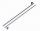On how many parts divide plane 6 parallels?
• A plane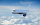A plane is flying at the rate of 350 mph after an eight hour flight is at its destination how far did the plane fly?